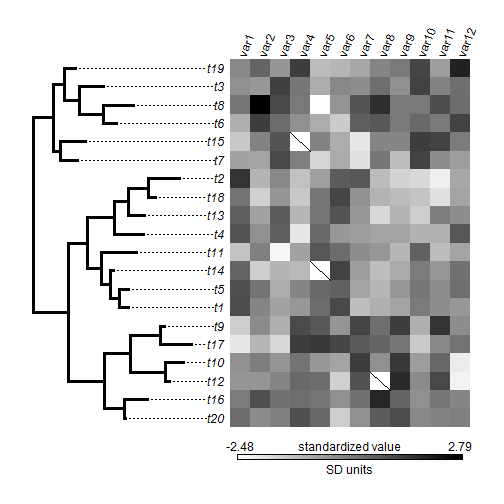## Thursday, February 4, 2016

### `phylo.heatmap` with missing values as `NA`

I just posted an update to `phylo.heatmap` that addresses a user request that the function permit missing values as `NA`. I did this first by just adding a bunch of `na.rm=TRUE` tags; however there remained a problem that in each instance that there was an `NA` in the data matrix to be plotted, the cell would be colored white. This is fine the color white is completely outside the range of plotted colors; however deceptively misleading if it is included, or at the margin, of this range!

My solution was to first identify the cells with `NA`s, and then draw a 'strikeout' line through each plotted cell, with white as the background. Here's my code to do so:

``````if(any(is.na(X))){
ii<-which(is.na(X),arr.ind=TRUE)
x.na<-seq(START,END,by=(END-START)/(ncol(X)-1))[ii[,2]]
y.na<-seq(0,1,by=1/(nrow(X)-1))[ii[,1]]
for(i in 1:length(x.na)){
xx<-x.na[i]+c(1/2,-1/2)*(END-START)/(ncol(X)-1)
yy<-y.na[i]+c(-1/2,1/2)*1/(nrow(X)-1)
lines(xx,yy)
}
}
``````

I also added the option, in response to two different user comments (1, 2) to turn off the plotted points at the end of each tip of the tree (even though I like that feature).

Here's a demo:

``````library(phytools)
colors<-colorRampPalette(colors=c("white","black"))(100)
phylo.heatmap(tree,X,colors=colors,standardize=TRUE,lwd=3,
pts=FALSE)
``````This same argument, `pts`, can also be used in `dotTree` and in the S3 plotting method for objects of class `"cophylo"`.

A version of phytools with these updates can be installed using devtools:

``````library(devtools)
install_github("liamrevell/phytools")
``````

The data & tree were simulated as follows:

``````tree<-rtree(n=20)
X<-fastBM(tree,n=12)
X[sample(1:(ncol(X)*nrow(X)),3)]<-NA ## random missing data
``````

1.Thanks, Liam! That works for me!

2.Thanks Liam for this function update.
Unfortunately I got a few error messages when following your instruction here to work with my tree and my trait matrix files.

When I execute
phylo.heatmap(mytree,mymatrix,colors=colors,standardize=TRUE,lwd=3,pts=FALSE)

I got
Error in X - matrix(rep(1, Ntip(tree)), Ntip(tree), 1) %*% colMeans(X, :
non-conformable arrays

When I execute
> phylo.heatmap(mytree,mymatrix,colors=colors,lwd=3,pts=FALSE)

I got
Error in X[cw\$tip.label, ] : subscript out of bounds

Thanks.

1.My best guess is that your matrix is either not the correct dimensions, not a matrix, or does not have row names corresponding to the tip labels of the tree. Do you know how to check these things?

2.Hi, please can you clarify what you mean by correct dimensions? I get the same error messages but as far as I can tell, the row names = tip labels, and I am using a matrix as input with my tree

3.Hi there!

First, thank you for putting together all of this code.

I'm trying to use phylo.heatmap but get the error:

Error in (2 - 0.5) * split/split + 0.5 - h :
non-numeric argument to binary operator

Any thoughts on what this might be?

Best,
Alex

1.Hi Alex. Not sure. Can you send me your saved workspace & the commands that generated the error? Thanks! Liam

2.The problem ended up being somehow associated with the function "phylogram" in h <- phylogram(tree, fsize=fsize,...), but removing some associated packages (e.g. mnormt, msm) & re-installing package fixed it.

Thanks again for your help and this package. I'm using this for studies of the human microbiome and we have a lot of tips - if you come up with a good way to get rid of the tip labels, I'd love to know!

Note: due to the very large amount of spam, all comments are now automatically submitted for moderation.